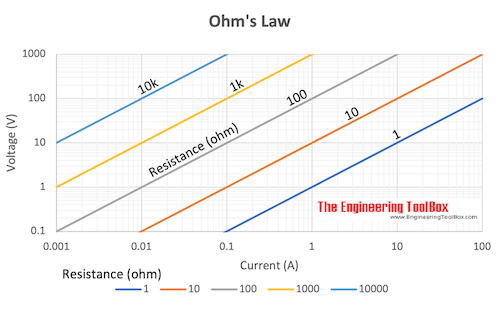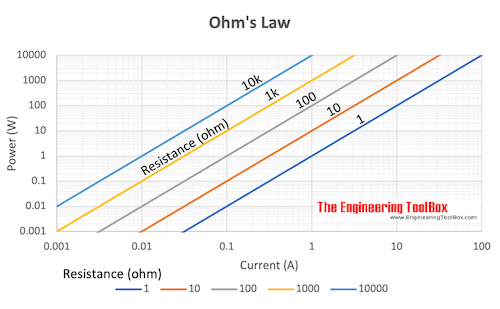Engineering ToolBox - Resources, Tools and Basic Information for Engineering and Design of Technical Applications!

# Electrical Formulas

## Commonly used electrical formulas like Ohms Law and more.

Common electrical units used in formulas and equations are:

• Volt - unit of electrical potential or motive force - potential is required to send one ampere of current through one ohm of resistance
• Ohm - unit of resistance - one ohm is the resistance offered to the passage of one ampere when impelled by one volt
• Ampere - units of current - one ampere is the current which one volt can send through a resistance of one ohm
• Watt - unit of electrical energy or power - one watt is the product of one ampere and one volt - one ampere of current flowing under the force of one volt gives one watt of energy
• Volt Ampere - product of volts and amperes as shown by a voltmeter and ammeter - in direct current systems the volt ampere is the same as watts or the energy delivered - in alternating current systems - the volts and amperes may or may not be 100% synchronous - when synchronous the volt amperes equals the watts on a wattmeter - when not synchronous volt amperes exceed watts - reactive power
• kiloVolt Ampere - one kilovolt ampere - kVA - is equal to 1000 volt amperes
• Power Factor - ratio of watts to volt amperes

### Electrical Potential - Ohm's Law

Ohm's law can be expressed as:

U = R I                        (1a)

U = P / I                      (1b)

U = (P R)1/2                (1c)Download and print Ohm's Law

### Electric Current - Ohm's Law

I = U / R                      (2a)

I = P / U                      (2b)

I = (P / R)1/2               (2c)

### Electric Resistance - Ohm's Law

R = U / I                     (3a)

R = U2/ P                    (3b)

R = P / I2                    (3c)

### Example - Ohm's law

A 12 volt battery supplies power to a resistance of 18 ohms.

I = (12 V) / (18 Ω)

= 0.67 (A)

### Electric Power

P = U I                      (4a)

P = R I2                    (4b)

P = U2/ R                  (4c)

where

P = power (watts, W, J/s)

U = voltage (volts, V)

I = current (amperes, A)

R = resistance (ohms, Ω)Download and print Ohm's Law

### Electric Energy

Electric energy is power multiplied with time:

W = P t                       (5)

where

W = energy (Ws, J)

t = time (s)

Alternative - power can be expressed

P = W / t                     (5b)

Power is consumption of energy by consumption of time.

#### Example - Energy lost in a Resistor

A 12 V battery is connected in series with a resistance of 50 ohm. The power consumed in the resistor can be calculated as

P = (12 V)2 / (50 ohm)

= 2.9 W

The energy dissipated in 60 seconds can be calculated

W = (2.9 W) (60 s)

= 174 Ws, J

= 0.174 kWs

= 4.8 10-5 kWh

#### Example - Electric Stove

An electric stove consumes 5 MJ of energy from a 230 V power supply when turned on in 60 minutes.

The power rating - energy per unit time - of the stove can be calculated as

P = (5 MJ) (106 J/MJ) / ((60 min) (60 s/min))

= 1389 W

= 1.39 kW

The current can be calculated

I = (1389 W) / (230 V)

= 6 ampere

### Electrical Motors

#### Electrical Motor Efficiency

μ = 746 Php / Pinput_w                 (6)

where

μ = efficiency

Php = output horsepower (hp)

Pinput_w = input electrical power (watts)

or alternatively

μ = 746 Php / (1.732 V I PF)                      (6b)

#### Electrical Motor - Power

P3-phase = (U I PF 1.732) / 1,000                    (7)

where

P3-phase = electrical power 3-phase motor (kW)

PF = power factor electrical motor

#### Electrical Motor - Amps

I3-phase = (746 Php) / (1.732 V μ PF)                  (8)

where

I3-phase = electrical current 3-phase motor (amps)

PF = power factor electrical motor

## Related Topics

• Electrical - Electrical units, amps and electrical wiring, wire gauge and AWG, electrical formulas and motors.

## Engineering ToolBox - SketchUp Extension - Online 3D modeling!

Add standard and customized parametric components - like flange beams, lumbers, piping, stairs and more - to your Sketchup model with the Engineering ToolBox - SketchUp Extension - enabled for use with the amazing, fun and free SketchUp Make and SketchUp Pro .Add the Engineering ToolBox extension to your SketchUp from the SketchUp Pro Sketchup Extension Warehouse!

Translate

## Privacy

We don't collect information from our users. Only emails and answers are saved in our archive. Cookies are only used in the browser to improve user experience.

Some of our calculators and applications let you save application data to your local computer. These applications will - due to browser restrictions - send data between your browser and our server. We don't save this data.

## Citation

• Engineering ToolBox, (2003). Electrical Formulas. [online] Available at: https://www.engineeringtoolbox.com/electrical-formulas-d_455.html [Accessed Day Mo. Year].

Modify access date.

. .

#### Scientific Online Calculator3 30